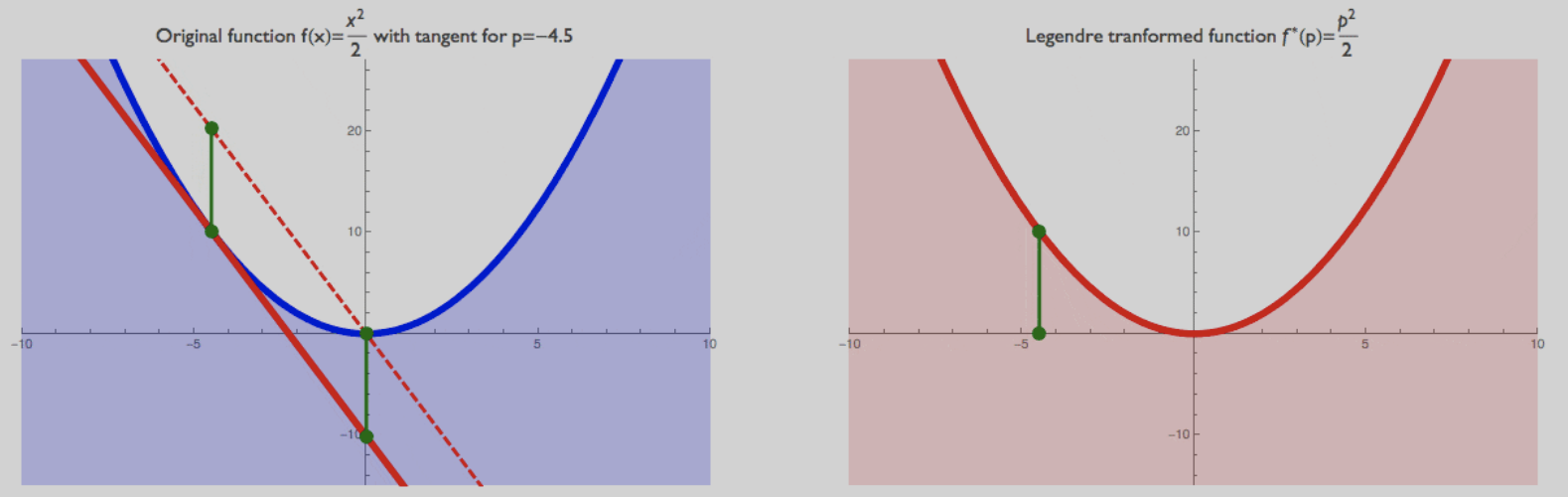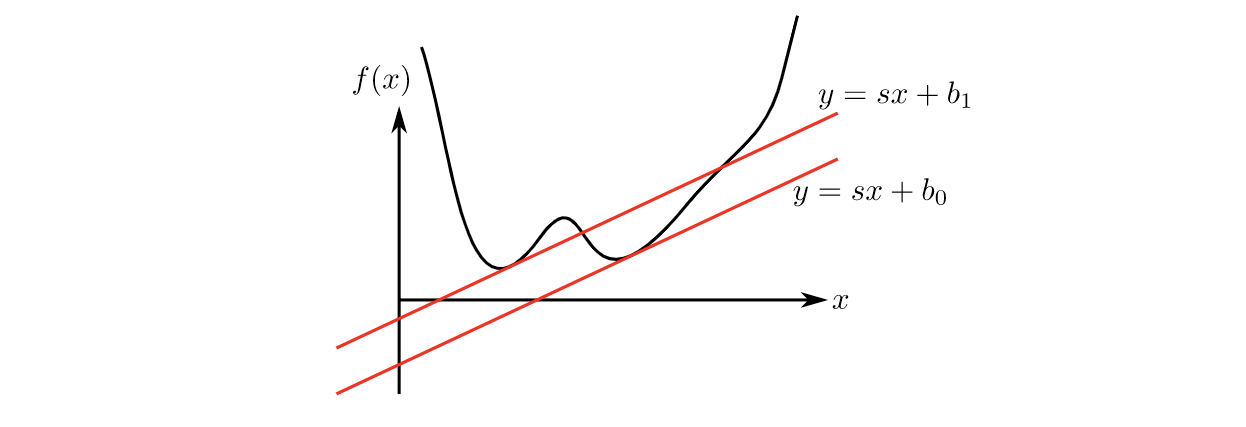N1H111SM's Miniverse

# Legendre Transform and Fenchel Conjugate

2020/06/17 ShareMaterials

# Legendre Transform

## Aim

Failed attempt. 一个非常直观的想法就是我们将 $x$ 用 $p$ 来表示，即 $x = \mathrm y ^{\prime -1}(p)$, 然后反向代回原来的函数 $\mathrm y$. 由此我们得到以下的transformed $\tilde{\mathrm y}$:

## Definition

Definition (Legendre Transformation). Legendre Transformation from a function $\mathrm y(x)$ to a new function $\mathrm y^\star (p)$ is defined as follow, where $p=\mathrm y^\prime (x)$ and no information is lost iff function $\mathrm y$ is convex (or concave, which is omitted in this blog):

## Properties

### Geometric interpretation### Inverse of Legendre Transformation

The Legendre Transformation of the Legendre Transformation of a function $\mathrm y$ is $\mathrm y$ itself, which is easy to prove.

# Fenchel ConjugateThis more general rule applies to non-differentiable or non-convex functions. So, when a line with slope $\mathbf s$ crosses $f(\mathbf x)$, we have:

and we want the smallest value of $b$ for all $x$. Then:

This is the Legendre-Fenchel transform, also known as convex conjugate. Note that now the transform is not reversible, i.e., you cannot get the original function by applying the transform to the transform. On the other hand, the transform of the transform is convex, even if the original function is not.